Скачать презентацию UTILITY and DEMAND UTILITY Utility is

a3dc784064200eb5173e3d38ca1c5df8.ppt

• Количество слайдов: 24UTILITY and DEMAND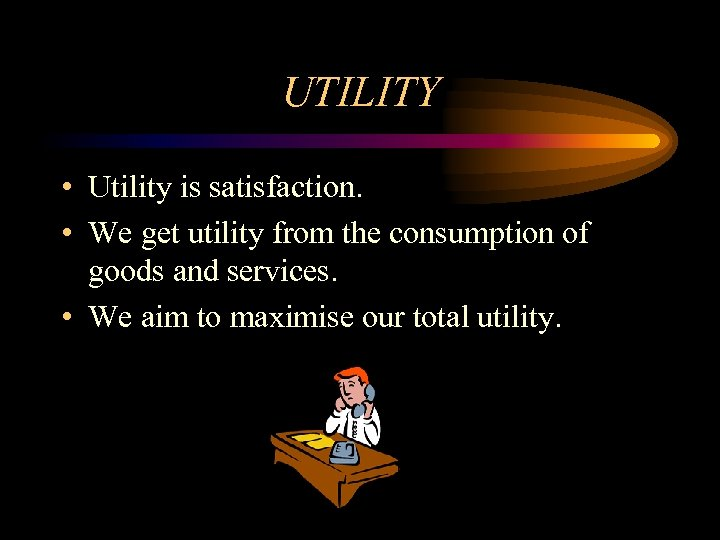UTILITY • Utility is satisfaction. • We get utility from the consumption of goods and services. • We aim to maximise our total utility.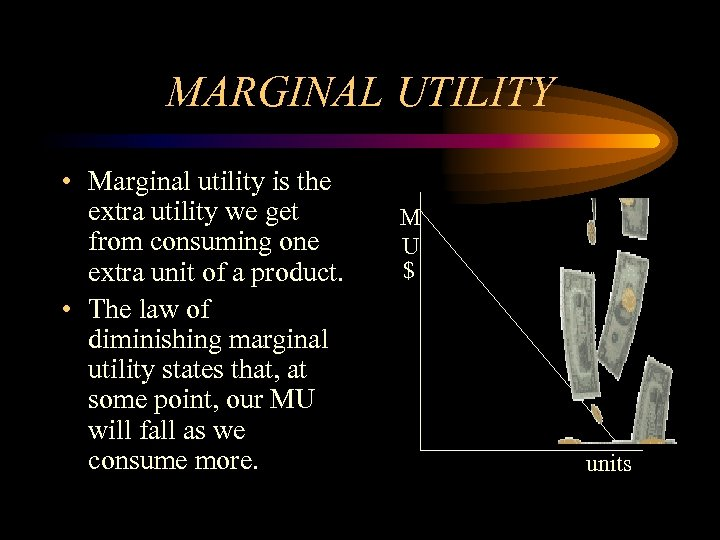MARGINAL UTILITY • Marginal utility is the extra utility we get from consuming one extra unit of a product. • The law of diminishing marginal utility states that, at some point, our MU will fall as we consume more. M U \$ units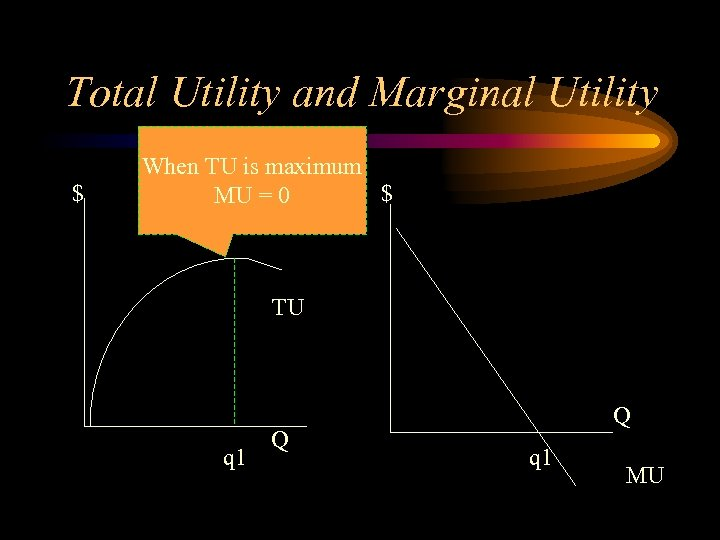Total Utility and Marginal Utility \$ When TU is maximum \$ MU = 0 TU q 1 Q Q q 1 MU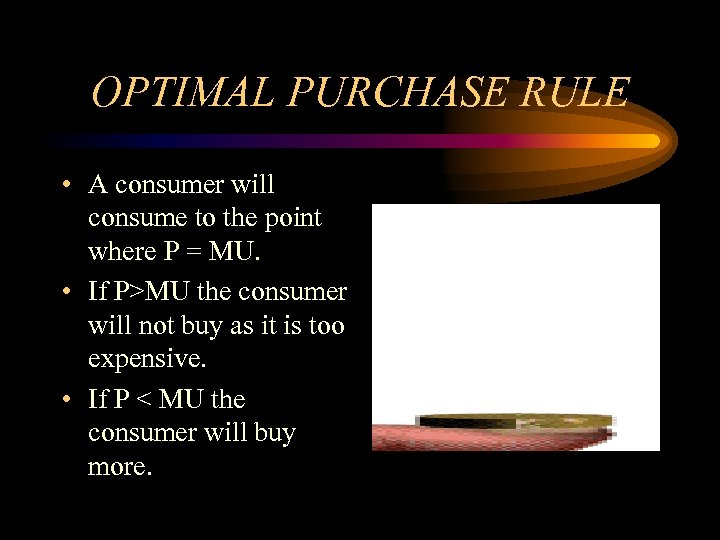OPTIMAL PURCHASE RULE • A consumer will consume to the point where P = MU. • If P>MU the consumer will not buy as it is too expensive. • If P < MU the consumer will buy more.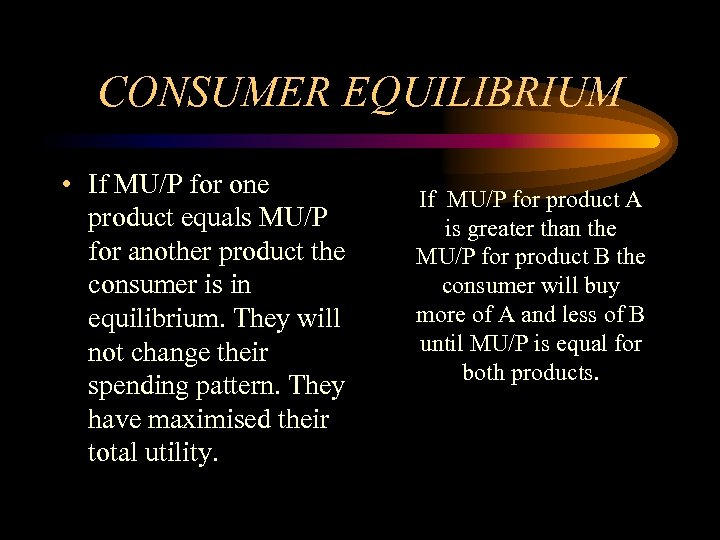CONSUMER EQUILIBRIUM • If MU/P for one product equals MU/P for another product the consumer is in equilibrium. They will not change their spending pattern. They have maximised their total utility. If MU/P for product A is greater than the MU/P for product B the consumer will buy more of A and less of B until MU/P is equal for both products.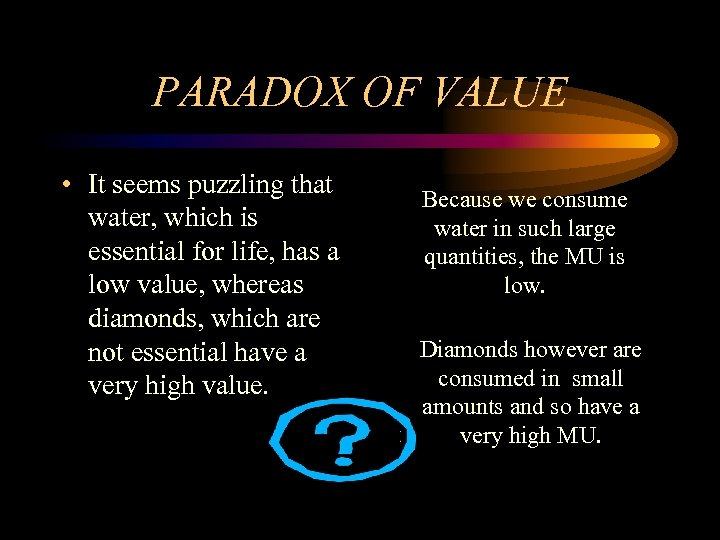PARADOX OF VALUE • It seems puzzling that water, which is essential for life, has a low value, whereas diamonds, which are not essential have a very high value. Because we consume water in such large quantities, the MU is low. Diamonds however are consumed in small amounts and so have a very high MU.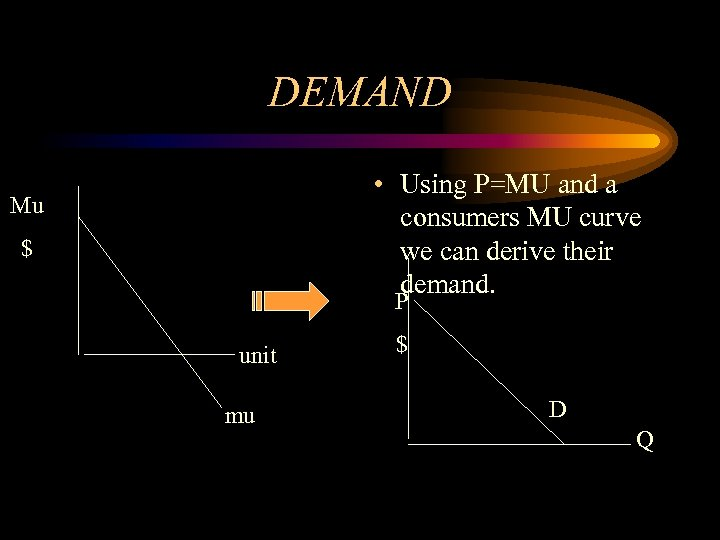DEMAND • Using P=MU and a consumers MU curve we can derive their demand. Mu \$ P unit mu \$ D Q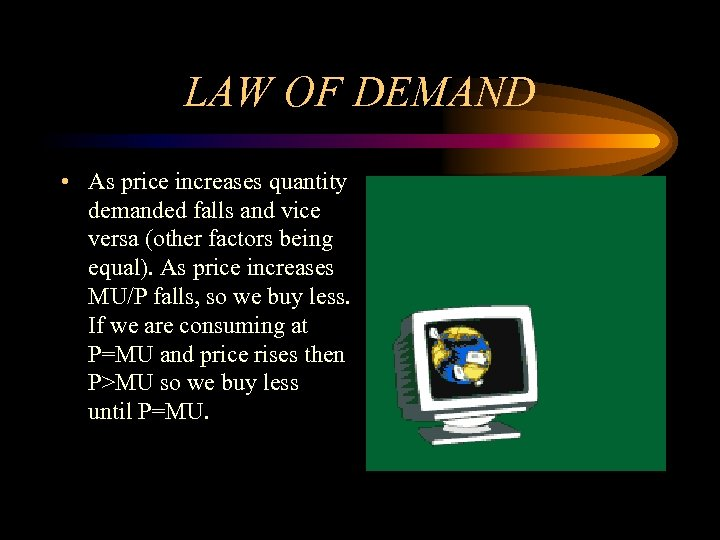LAW OF DEMAND • As price increases quantity demanded falls and vice versa (other factors being equal). As price increases MU/P falls, so we buy less. If we are consuming at P=MU and price rises then P>MU so we buy less until P=MU.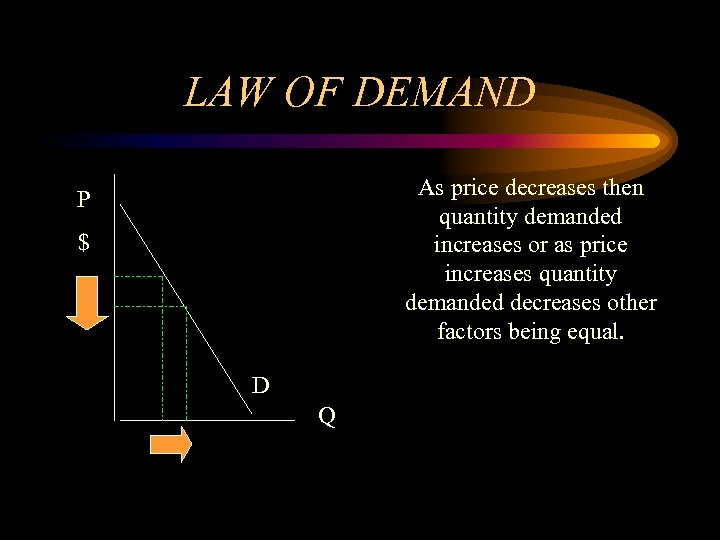LAW OF DEMAND As price decreases then quantity demanded increases or as price increases quantity demanded decreases other factors being equal. P \$ D Q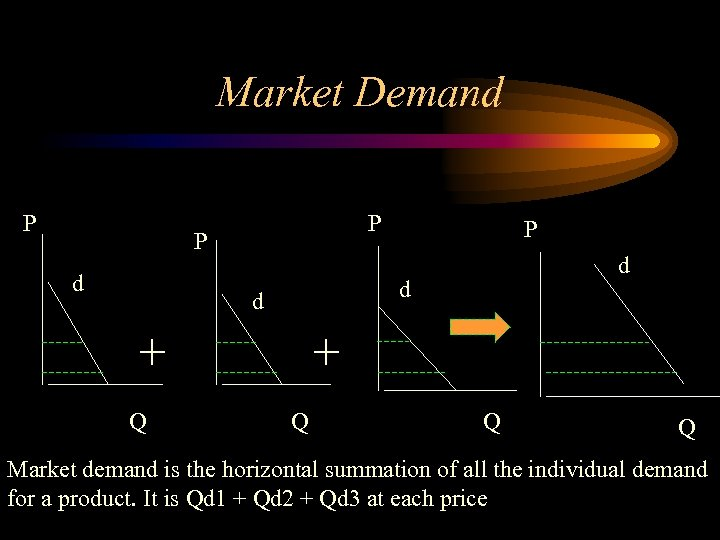Market Demand P P P d d + Q P + Q Q Q Market demand is the horizontal summation of all the individual demand for a product. It is Qd 1 + Qd 2 + Qd 3 at each price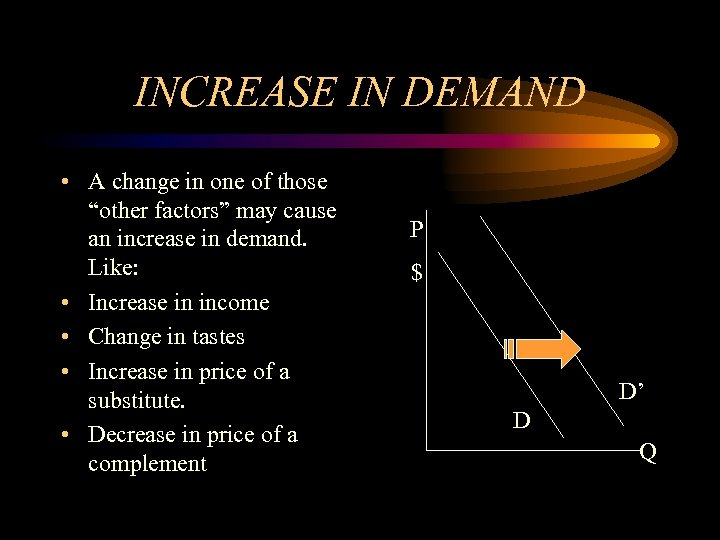INCREASE IN DEMAND • A change in one of those “other factors” may cause an increase in demand. Like: • Increase in income • Change in tastes • Increase in price of a substitute. • Decrease in price of a complement P \$ D’ D Q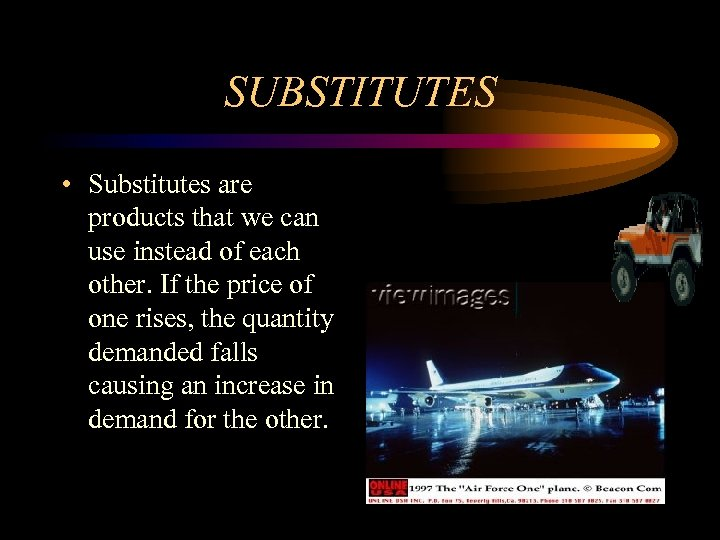SUBSTITUTES • Substitutes are products that we can use instead of each other. If the price of one rises, the quantity demanded falls causing an increase in demand for the other.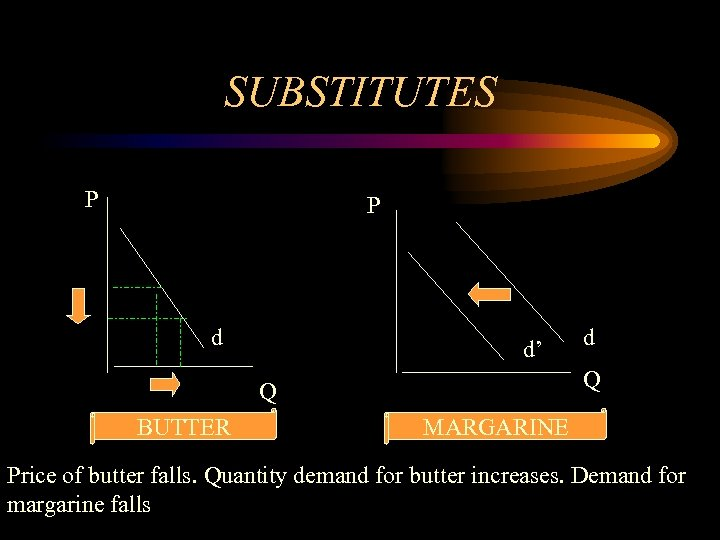SUBSTITUTES P P d d’ Q Q BUTTER d MARGARINE Price of butter falls. Quantity demand for butter increases. Demand for margarine fallsCOMPLEMENTS • Complements are products that we usually use together. If the price of one rises, quantity demanded falls causing a decrease in the demand for the other.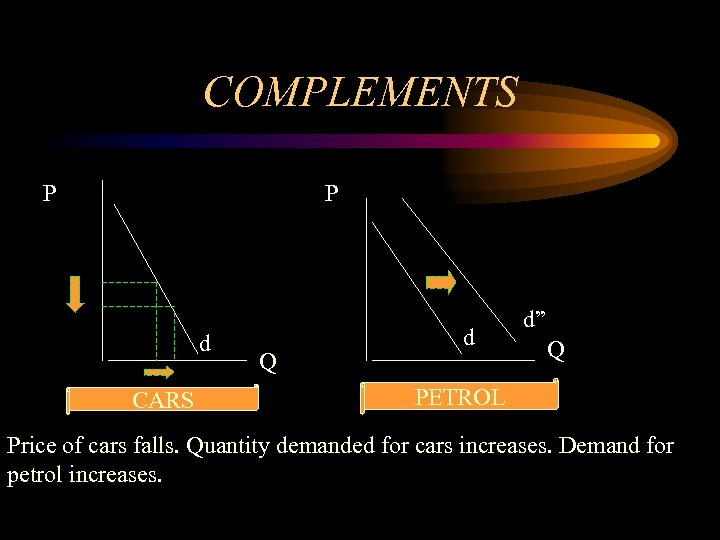COMPLEMENTS P P d CARS Q d d” Q PETROL Price of cars falls. Quantity demanded for cars increases. Demand for petrol increases.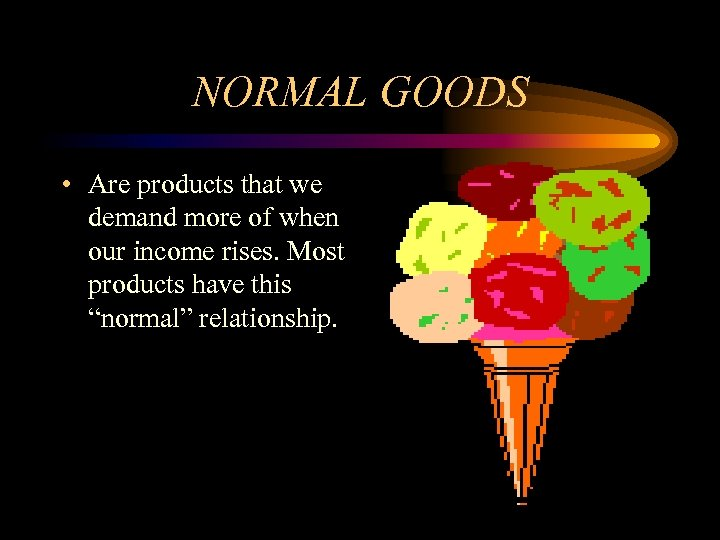NORMAL GOODS • Are products that we demand more of when our income rises. Most products have this “normal” relationship.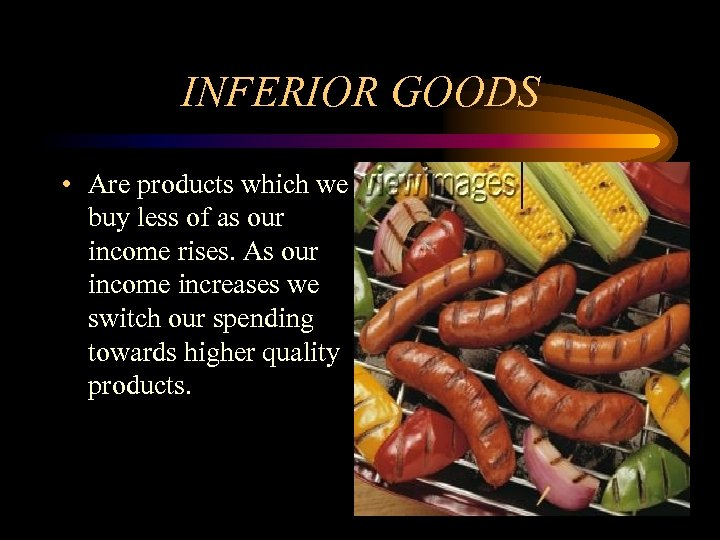INFERIOR GOODS • Are products which we buy less of as our income rises. As our income increases we switch our spending towards higher quality products.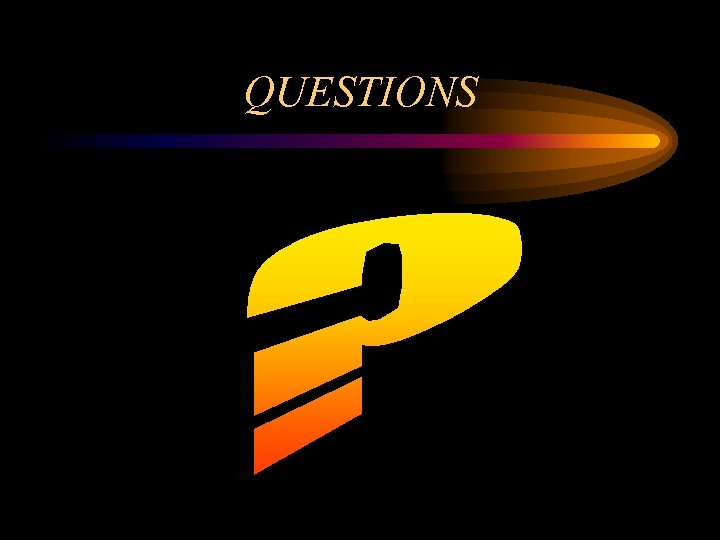QUESTIONS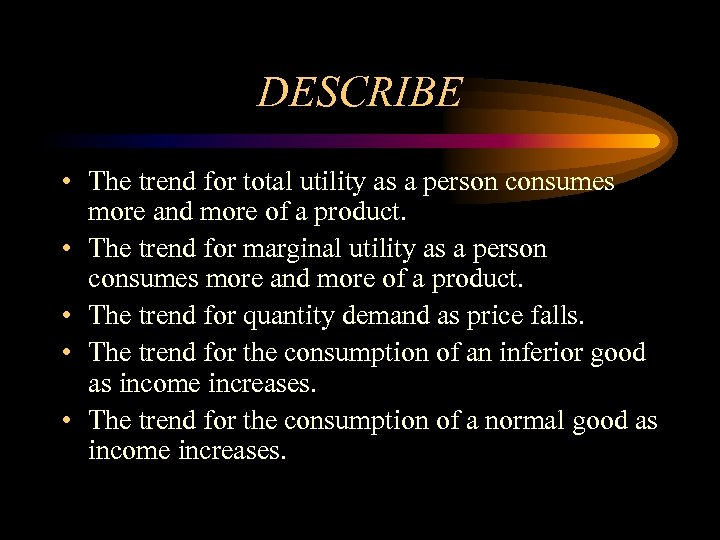DESCRIBE • The trend for total utility as a person consumes more and more of a product. • The trend for marginal utility as a person consumes more and more of a product. • The trend for quantity demand as price falls. • The trend for the consumption of an inferior good as income increases. • The trend for the consumption of a normal good as income increases.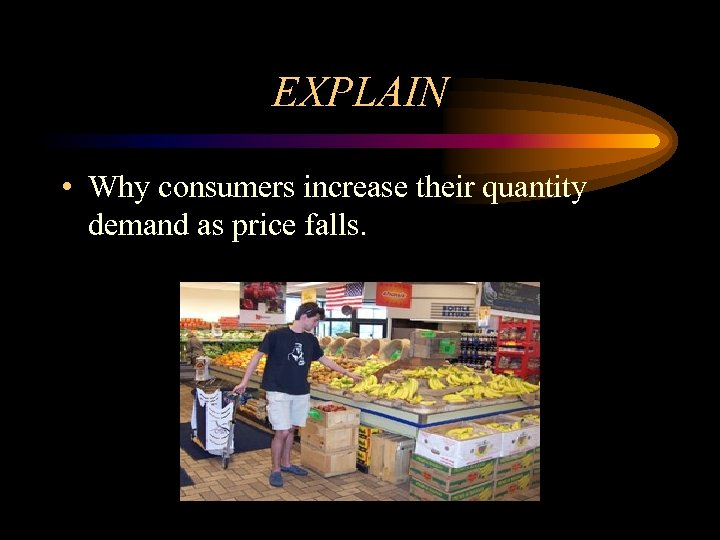EXPLAIN • Why consumers increase their quantity demand as price falls.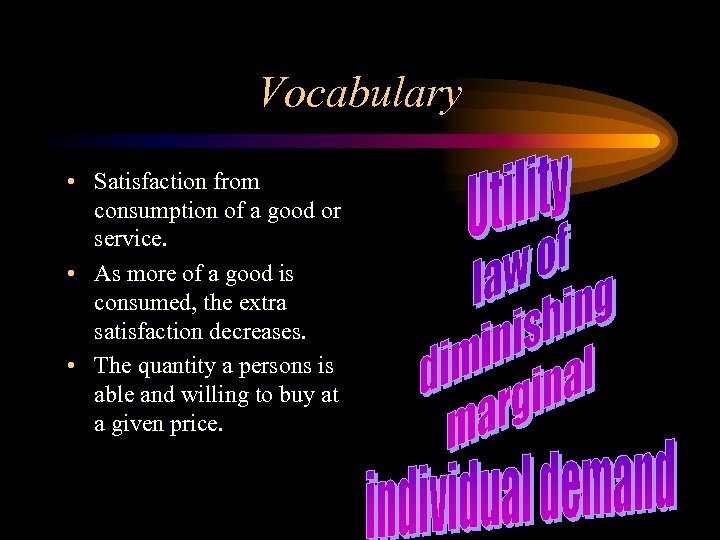Vocabulary • Satisfaction from consumption of a good or service. • As more of a good is consumed, the extra satisfaction decreases. • The quantity a persons is able and willing to buy at a given price.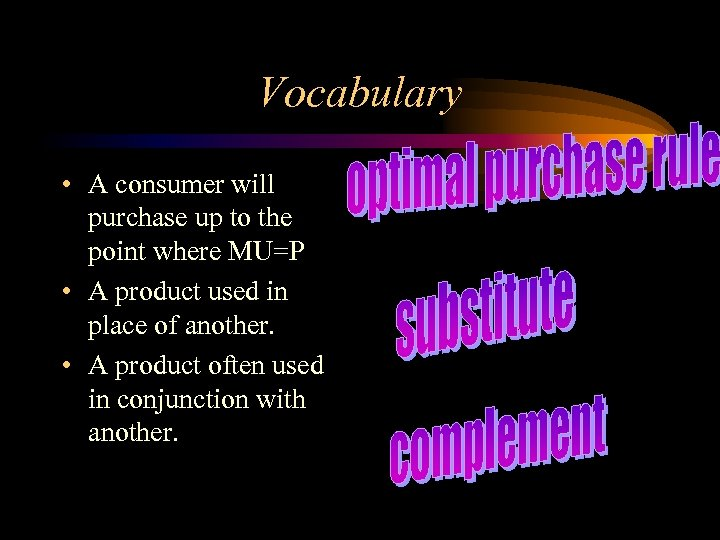Vocabulary • A consumer will purchase up to the point where MU=P • A product used in place of another. • A product often used in conjunction with another.